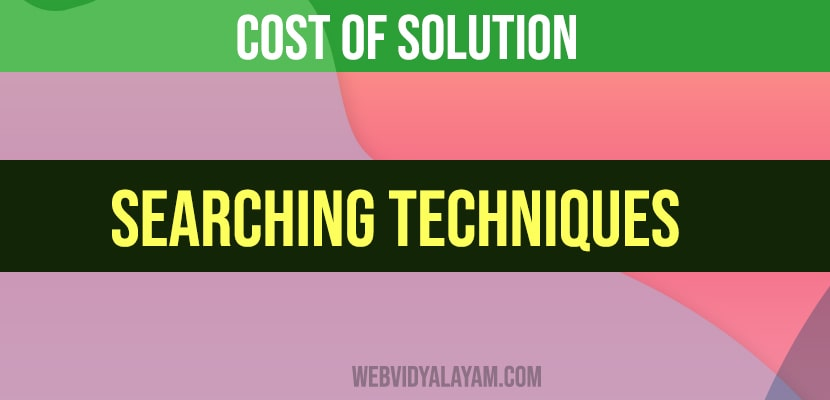# Cost Of Solution

1.Cost of  solution is the sum of the arc costs on the solution path.

• If all arcs have the same (unit) cost, then the solution cost is just the length of solution (number of steps/state transitions).then the solution cost is just the length of the solution or the number of steps or state transitions that is involved.

State space search  under such scenario is the process of searching through a state space for a solution, by making explicit a sufficient portion of an implicit state space graph.

Note: here we are generating the complete graph for finding a solution of problem solving. We keep on generating the graph as we keep on exploring for the solution.

In case of large state spaces, it is not possible in practical to represent the whole space.

1.Initially V= {S} , where S is the start node

•  When S is expanded its successors are generated and those nodes are added to the set V and the associated arcs are added to the set E.

• This process goes on until a goal node is reached.

So, Instead of having the whole state  space graph as it is explicitly generated and then looking for a solution, the idea is to make explicit a sufficient portion of this as I go on expanding for nodes.

And every node I expand  have to answer a question that whether it is my goal node ; if the answer is no then  getting the successor nodes and the process must  be continued  until the goal node is reached.

In general, from every node, there are many possible paths that have its partial  as prefix.